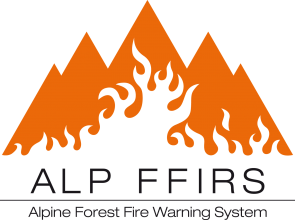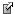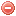# FrameIn the frame of the project ALPFFIRS, the Swiss federal institute of forest, snow and landscape research WSL, with the collaboration of the project partners, has set up this wiki on fire weather indices.
The aim of this wiki is to provide a public reference information on the mostly used fire weather indices, with detailed informations on indices purpose, applicability (validity, starting conditions, ...), implementations (modifications) and an exhaustive literature.
At the same time the WSL is developing an open source software application for the calculation of the most used fire weather indices.

# Fire weather indices

Better understanding fire activity has always been a major issue for foresters and ecologists. Although the relative importance of factors such as weather, forest composition, and anthropogenic influences on forest fire activity is disputed (e.g. Cumming 2001), the prevailing paradigm suggests that climate and weather are the most important determinants of forest fires (e.g. Carcaillet et al. 2001, Ryan 2002, Whitlock et al. 2003). In particular, weather conditions have been found to be especially important on short time scales, i.e. daily conditions (Bessie and Johnson 1995).
Accordingly, a particular emphasis has been put on how to predict fire ignition and behavior using fire indices that depend on climate and weather variables. Thus previous research has focused on describing fire weather conditions, and integrating different meteorological variables into fire indices. These efforts resulted in a wide array of fire danger rating systems and indices that can be used to assess forest fire hazard (e.g. Munger 1916, Nesterov 1949, Keetch and Byram 1968, Haines et al. 1983, Van Wagner 1987, Goodrick 2002).
These various fire indices differ regarding many aspects. Some of them not only include relationships between weather conditions and fire activity but also between fire activity and soil moisture and fuel properties. Moreover, they are meant to predict distinct elements of fire activity, such as fire ignition, spread, or intensity. For instance, some very simple indices intending to assess fire ignition probability only consider day-to-day weather conditions. Other more complex indices (similarly to drought indices) are cumulative and consider water content in soil or fuels over longer periods, being thus suitable for predicting fire intensity or spread.
Most fire indices are empirical models. Therefore, their validity is often limited to the specific type of climate or vegetation where they were developed. Comparative studies evaluated fire indices developed in different countries by applying them in different other regions in Europe (e.g Viegas et al. 1999 in the Mediterranean basin, and Weibel 2009 in the Swiss Alps). The predictive ability (number of fire days, number of fires, area burned) of the fire indices was found to differ according to ignition type (natural/anthropogenic), season and the region for which the index was applied. These studies concluded that the predictive value of individual indices varies greatly depending on the environmental conditions under which they are applied. This underlines the fact that transferring fire indices from one region to another should only be performed with caution, and calls for careful analyses to evaluate the suitability of fire indices when applied in specific regions.
In the present study, we considered and analyzed some twenty of the most well-known past and contemporary fire indices developed in North America (Canadian FWI System, Fosberg index, KBDI, Munger index and NFDRS), Europe (Angström, Baumgartner, Carrega, Käse-M68, Orieux, Nesterov and Drouet-Sol indices) and Australia (McArthur Mark 5 and Sharples indices). We then evaluated their suitability for predicting fire danger using datasets from several European countries. This study should therefore provide a decision tool allowing forest managers and scientists to select fire indices for predicting adequately fire danger in their respective regions.

Bessie and Johnson (1995)
Carcaillet et al. (2001)
Cumming (2001)
Goodrick (2002)
Haines et al. (1983)
Keetch and Byram (1968)
Munger (1916)
Nesterov (1949)
Ryan (2002)
Van Wagner (1987)
Viegas et al. (1999)
Weibel (2009)
Whitlock et al. (2003)

### Bibliography SearchNo results!

No results were found. Are you sure you searched for a tag?

### Symbols

 Variable Description Unit $$T$$ air temperature °C $$T_{dew}$$ dew point temperature °C $$H$$ air humidity % $$P$$ rainfall mm $$U$$ windspeed m/s $$w$$ days since last rain (or rain above threshold) d $$rr$$ days with consecutive rain d $$\Delta t$$ time increment d $$\Delta{e}$$ vapor pressure deficit kPa $$e_s$$ saturation vapor pressure kPa $$e_a$$ actual vapor pressure kPa $$p_{atm}$$ atmospheric pressure kPa $$PET$$ potential evapotranspiration mm/d $$r$$ soil water reserve mm $$r_s$$ surface water reserve mm $$EMC$$ equilibrium moisture content % $$DF$$ drought factor - $$N$$ daylight hours hr $$D$$ weighted 24-hr average moisture condition hr $$\omega$$ sunset hour angle rad $$\delta$$ solar declination rad $$\varphi$$ latitude rad $$Cc$$ cloud cover Okta $$J$$ day of the year (1..365/366) - $$I$$ heat index - $$R_n$$ net radiation MJ⋅m-2⋅d-1 $$R_a$$ daily extraterrestrial radiation MJ⋅m-2⋅d-1 $$R_s$$ solar radiation MJ⋅m-2⋅d-1 $$R_{so}$$ clear-sky solar radiation MJ⋅m-2⋅d-1 $$R_{ns}$$ net shortwave radiation MJ⋅m-2⋅d-1 $$R_{nl}$$ net longwave radiation MJ⋅m-2⋅d-1 $$\lambda$$ latent heat of vaporization MJ/kg $$z$$ elevation m a.s.l. $$d_r$$ inverse relative distance Earth-Sun - $$\alpha$$ albedo or canopy reflection coefficient - $$\Delta$$ slope of the saturation vapor pressure curve kPa/°C $$Cc$$ cloud cover eights $$ROS$$ rate of spread m/h $$RSF$$ rate of spread factor - $$WF$$ wind factor - $$WRF$$ water reserve factor - $$FH$$ false relative humidity - $$FAF$$ fuel availability factor - $$PC$$ phenological coefficient -

 Suffix Description $$-$$ mean / daily value $$_{max}$$ maximum value $$_{min}$$ minimum value $$_{12}$$ value at 12:00 $$_{13}$$ value at 13:00 $$_{15}$$ value at 15:00 $$_{m}$$ montly value $$_{y}$$ yearly value $$_{f/a}$$ value at fuel-atmosphere interface $$_{dur}$$ duration $$_{soil}$$ value at soil level

 Constant Description $$e$$ Euler's number $$\gamma$$ psychrometric constant $$G_{SC}$$ solar constant $$\sigma$$ Stefan-Bolzmann constant